Next: Fluid Equations in Spherical Up: Mathematical Models of Fluid Previous: Fluid Equations in Cartesian

# Fluid Equations in Cylindrical Coordinates

Let us adopt the cylindrical coordinate system, (,,). Making use of the results quoted in Section C.3, the components of the stress tensor are(1.142)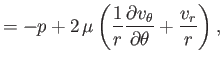(1.143)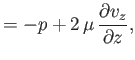(1.144)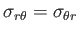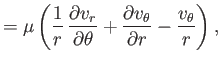(1.145)(1.146)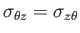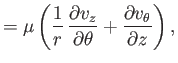(1.147)

whereas the equations of compressible fluid flow become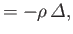(1.148)(1.149)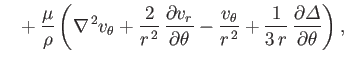(1.150)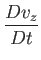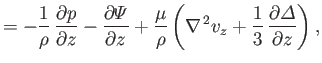(1.151)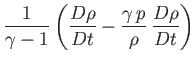(1.152)

where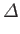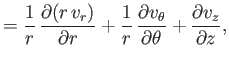(1.153)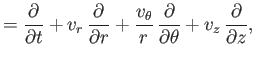(1.154)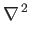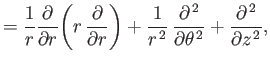(1.155)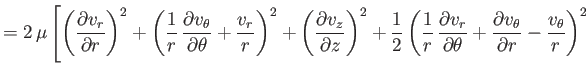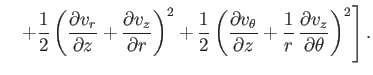(1.156)Next: Fluid Equations in Spherical Up: Mathematical Models of Fluid Previous: Fluid Equations in Cartesian
Richard Fitzpatrick 2016-03-31